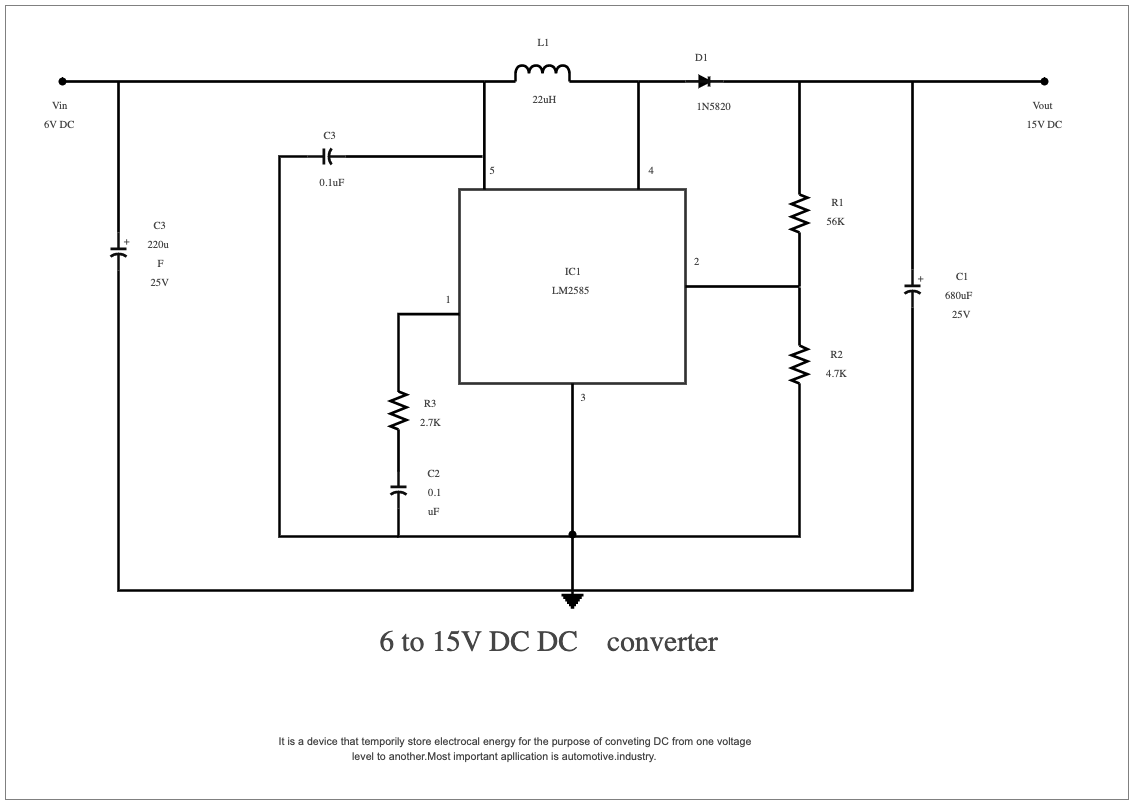Template Community / 6 Volt DC to 15 Volt DC Converter Circuit Diagram

# 6 Volt DC to 15 Volt DC Converter Circuit DiagramElectrical Drawing
Published on 2022-12-22
Edit OnlineA 6-volt DC to 15-volt DC converter circuit diagram is a schematic representation of the electrical components and wiring needed to transform a low-voltage direct current power source into a higher-voltage one. It usually includes the components required, such as resistors, capacitors, transistors, and switches. The circuit diagram also illustrates the connections between each component for the effective conversion of energy. For applications that require varying voltage outputs, a 6 Volt DC to 15 Volt DC converter circuit can be used. This device stores electrical energy temporarily and converts it from one voltage level to another. Its most common use is in the automotive industry, where it ensures the correct voltage output when starting a vehicle. The circuit diagram for this kind of converter can be found online and provides a quick and easy way of setting up the necessary components to get the desired voltage output. Easily recreate similar elaborated diagrams using the free template and systems provided by EdrawMax.
Tag
circuit diagram
Report
1
15PostRecommended Templates